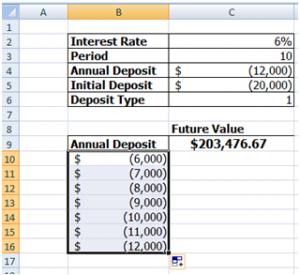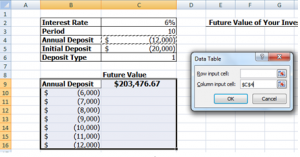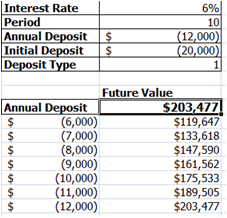## Data Tables can help you save time in Excel data analysisWhile working in Microsoft Excel on data analysis, there are a handful of built-in analysis tools available.  If you’re interested in studying the effect that a range of values has on the formula, you should consider setting up a data table.

Let’s consider an investment analysis example to start.  Suppose that you want to see the future value of an investment with the annual deposit varying between \$6,000 and \$11,000.  You could enter these values in a row or column, and then create the formulas.  That would take a while and we all got things to do.

Setting up a data table is much easier and less time consuming.  More importantly, using a data table you can harness the power of a What-If analysis.  The data table can be based on the row or column input cell data.

## How does a Data Table work?A Data Table is a complex “what-if” analysis tool.  In standard formulas, we might reference a cell and treat that reference like a variable.  However, unlike a traditional variable, a data table uses an array variable in the formula for the interior of the data table.

A traditional variable stores one value in one variable.  For example, x=10 means that the value of 10 is stored as ‘x’.

An array is used to store multiple values within one variable in a serialized fashion.

In this example, we want to store the values of the annual deposit in one array variable.  The different values will be swapped into the future value function for each row, and the output value will be filled in quickly and easily.In a data table, an array is storing the cell references that hold the values to create the final result.  This means that you can’t change or delete individual elements of the array.

If you want to change the results of the data table array, you need to select the entire data table (B9:C16) and then re-run the data table command again.  If you just want to delete the results, you must first select the entire array (C10:C16) and then delete it.

In the end, the data table can create all of the future value answers in one easy step.  If you are interested in more practical application of the data table, learn more about what-if analysis tools.

## Interested in learning more about Excel training?

Fill out the following form and we can help design a custom training plan for you and your group: